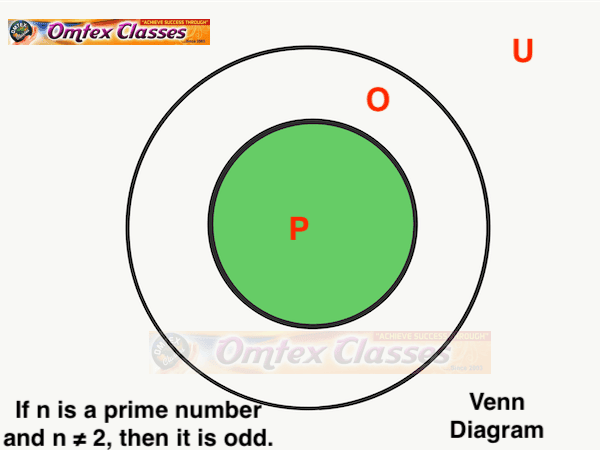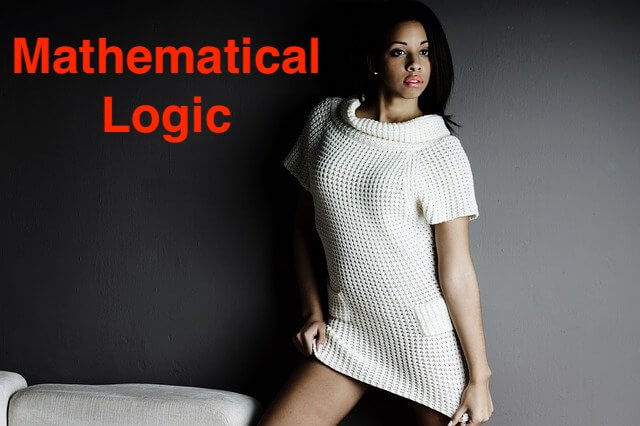Represent the following statement by the Venn diagram. If n is a prime number and n ≠ 2, then it is odd.

EXERCISE 1.10Q 3.3  PAGE 27
Exercise 1.10 | Q 3.3 | Page 27

Represent the following statement by the Venn diagram.

If n is a prime number and n ≠ 2, then it is odd.

SOLUTION

Let, U : The set of all real numbers.
P : The set of all prime numbers n and n ≠ 2.
O : The set of all odd numbers.The above Venn diagram represents the truth of the given statement i.e., P ⊂ O.